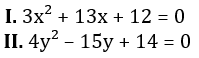SBI PO Quantitative Aptitude Quiz

Here, we are providing SBI PO Mains Study plan as there is left not enough time to deal in details. The questions asked in the quantitative aptitude section are calculative and very time-consuming. But once dealt with proper strategy, speed, and accuracy, this section can get you the maximum marks in the examination. Following is the Quantitative Aptitude quiz to help you practice with the best of latest pattern questions

Q1. Out of Rs. 50000, that a man has, he lends Rs. 8000 at 11/2% per annum simple interest and Rs. 24000 at 6% per annum simple interest. He lends the remaining money at a certain rate of simple interest so that he gets total annual simple interest of Rs. 3680. The rate of simple interest per annum, at which the remaining money is lent, is
5%
7%
10%
12%
15%

Q2. A committee of five persons is formed from four boys and five girls. Find the probability that there are at least two girls in the committee?
119/126
53/126
121/126
113/126
None of these

Q3. Abhinav leaves Mumbai at 6 am and reaches Bangalore at 10 am and Praveen leaves Bangalore at 8 am and reaches Mumbai at 11 : 30 am. At what time do they cross each other?
10 am
8 : 32 am
8 : 56 am
9 : 20 am
None of these

Q4. A sum is divided between A and B in the ratio of 1 : 2. A purchased a car from his part, which depreciatesper annum and B deposited his amount in a bank, which pays him 20% interest per annum compounded annually. By what percentage will the total sum of money increase than the original sum after two years due to this investment pattern (approximately)?
20%
26.66%
30%
25%
33.33%

Q5. Aniruddh can finish a job in 20 days. Ritika and Sakshi together can finish the same job in 10 days. If ratio of efficiency of Ritika and Sakshi is 1:3 respectively, then find the time taken by all three to complete the same job working together.
20/3 days
5 days
17/3 days
23/3 days
6 days

Q6. Two containers contain honey and milk in the ratio 7:3 and 2:3 respectively. In what ratio should the contents of the containers be mixed such that the final ratio of honey and milk in the resultant solution becomes 23:17.
5 : 3
7 : 5
4 : 3
11 : 9
7 : 6

Q7. The curved surface area of a cylinder is equal to the curved surface area of a cone. If radius of both is equal and radius of cone is twice of its height, then find the ratio of height of cylinder to that of cone.
2: √5
1: 2
√5: 2
√3: 1
√5: 3

Q8. A man bought a scooter and a car. He sold all of them at 30% profit. Scooter is sold at 10% profit. Cost price of scooter is 1/10 of the cost of car. Marked price of the car is Rs. 4,50,000. If he bought scooter at a discount of 20% on marked price and car at a discount of 10% on marked price then, what will be the ratio of marked price of scooter to the selling price of the car.
25/264
25/268
35/260
34/260
None of these

Q9. Quantity 1: Height of the tank if the volume of cylindrical tank is 12320 cm³. Its radius and height are in the ratio of 7 : 10 respectively.
Quantity 2: Level of kerosene in the jar. A conical vessel of base radius 2 cm and height 3 cm is filled with kerosene. This liquid leak through a hole in the bottom and collects in a cylindrical jar of radius 2 cm.

Quantity I > Quantity II
Quantity I < Quantity II
Quantity I ≥ Quantity II
Quantity I ≤ Quantity II
Quantity I = Quantity II or No relation

Q10. A man who swims 48m/minute in still water, swims 200m against the current and 200m with the current. The difference between those two times is 10 minutes. Quantity 1: speed of current.
Quantity 2: Speed of a man who completes 3 rounds of a circular path of radius 49 m in 14 minutes.

Quantity I > Quantity II
Quantity I < Quantity II
Quantity I ≥ Quantity II
Quantity I ≤ Quantity II
Quantity I = Quantity II or No relation

Directions (11-15): In each of these questions, two equations (I) and (II) are given. You have to solve both the equations and give answer

Q11.if x > y
if x ≥ y
if x < y
if x ≤ y
if x = y or no relation can be established between x and y.

Q12.if x > y
if x ≥ y
if x < y
if x ≤ y
if x = y or no relation can be established between x and y.

Q13.if x > y
if x ≥ y
if x < y
if x ≤ y
if x = y or no relation can be established between x and y.

Q14.if x > y
if x ≥ y
if x < y
if x ≤ y
if x = y or no relation can be established between x and y.

Q15.if x > y
if x ≥ y
if x < y
if x ≤ y
if x = y or no relation can be established between x and y.

You May also like to Read:#### 1 comment:

1.8th question ka answer would be 5:48